Courses

# RD Sharma Solutions - Ex-16.3, Congruence, Class 7, Math Class 7 Notes | EduRev

## Class 7: RD Sharma Solutions - Ex-16.3, Congruence, Class 7, Math Class 7 Notes | EduRev

The document RD Sharma Solutions - Ex-16.3, Congruence, Class 7, Math Class 7 Notes | EduRev is a part of the Class 7 Course RD Sharma Solutions for Class 7 Mathematics.
All you need of Class 7 at this link: Class 7

#### Question 1:

By applying SAS congruence condition, state which of the following pairs (Fig. 28) of triangles are congruent. State the result in symbolic form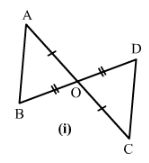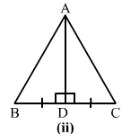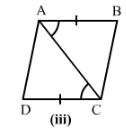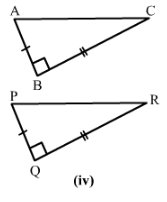1) We have OA = OC and OB = OD and∠AOB =∠COD which are vertically opposite angles.
Therefore by SAS condition, △AOC ≅ △BOD.

2) We have BD = DC

3)   We have AB = DC
∠∠ABD = ∠CDB and BD = DB
Therefore by SAS condition, △ABD ≅ △CBD.

4)  We have BC = QR
∠ABC =∠PQR = 90°
and AB = PQ
Therefore by SAS condition, △ABC ≅ △PQR.

#### Question 2:

State the condition by which the following pairs of triangles are congruent.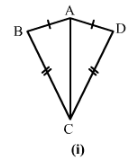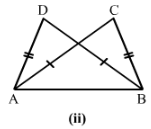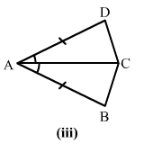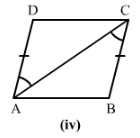BC = CD
and AC = CA

2) AC = BD
AD = BC and AB = BA
Therefore by SSS condition, △ABD≅△BAC.

∠BAC=∠DAC∠BAC=∠DAC
and AC = AC
Therefore by SAS condition, △BAC≅△DAC.

∠ DAC = ∠BCA
and AC = CA

#### Question 3:

In Fig. 30, line segments AB and CD bisect each other at O. Which of the following statements is true?
(i) ∆ AOC ≅ ∆ DOB
(ii) ∆ AOC ≅ ∆ BOD
(iii) ∆ AOC ≅ ∆ ODB.
State the three pairs of matching parts, yut have used to arive at the answer.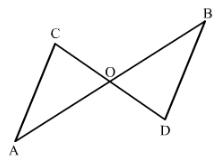We have AO = OB.
And CO = OD

Also ∠AOC = ∠BOD
Therefore by SAS condition, △AOC ≅ △BOD.

Therefore, statement (ii) is true.

#### Question 4:

Line-segments AB and CD bisect each other at OAC and BD are joined forming triangles AOC and BOD. State the three equality relations between the parts of the two triangles, that are given or otherwise known. Are the two triangles congruent? State in symbolic form. Which congruence condition do you use?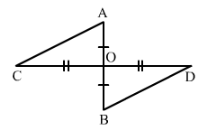We have AO = OB and CO = OD since AB and CD bisect each other at O.

Also ∠AOC = ∠BOD since they are opposite angles on the same vertex.
Therefore by SAS congruence condition,

△AOC≅△BOD

#### Question 5:

∆ ABC is isosceles with ABAC. Line segment AD bisects ∠A and meets the base BC in D.
(ii) State the three pairs of matching parts used to answer (i).
(iii) Is it true to say that BDDC?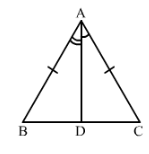(i) We have AB = AC (given)
Therefore by SAS condition of congruence, △ABD≅△ACD

(iii) Now△ABD≅△ACD  therefore by c.p.c.t BD = DC.

#### Question 6:

In Fig. 31, ABAD and ∠BAC = ∠DAC.
(i) State in symbolic form the congruence of two triangles ABC and ADC that is true.
(ii) Complete each of the following, so as to make it true:
(a) ∠ABC = ........
(b) ∠ACD = ........
(c) Line segment AC bisects ..... and .....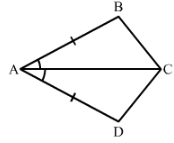∠BAC =∠DAC  (given)
AC = CA (common)
Therefore by SAS conditionof congruency, △ABC ≅△ADC

∠ACD =∠ACB (c.p.c.t)

#### Question 7:

In Fig. 32, AB || DC and ABDC.
(i) Is ∆ ACD ≅ ∆ CAB?
(ii) State the three pairs of matching parts used to answer (i).
(iii) Which angle is equal to ∠CAD?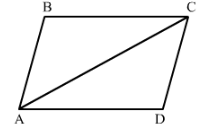(i) Yes △ACD≅△CAB by SAS condition of congruency.
(ii) We have used AB = DC, AC = CA and ∠DCA=∠BAC.
(iii) ∠CAD =∠ACB  since the two triangles are congruent.
(iv) Yes, this follows from AD∥∥BC as alternate angles are equal.If alternate angles are equal the lines are parallel.

The document RD Sharma Solutions - Ex-16.3, Congruence, Class 7, Math Class 7 Notes | EduRev is a part of the Class 7 Course RD Sharma Solutions for Class 7 Mathematics.
All you need of Class 7 at this link: Class 7Use Code STAYHOME200 and get INR 200 additional OFF Use Coupon Code
All Tests, Videos & Notes of Class 7: Class 7

### Top Courses for Class 7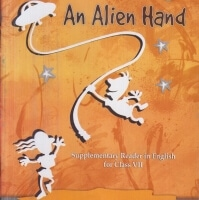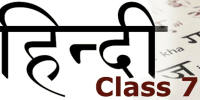## RD Sharma Solutions for Class 7 Mathematics

97 docs

### Top Courses for Class 7Track your progress, build streaks, highlight & save important lessons and more!

,

,

,

,

,

,

,

,

,

,

,

,

,

,

,

,

,

,

,

,

,

,

,

,

,

,

,

,

,

,

;